Let us first understand the concept of thread in computer architecture.

In computing, a process is an instance of a computer program that is being executed. Any process has 3 basic components:

• An executable program.
• The associated data needed by the program (variables, work space, buffers, etc.)
• The execution context of the program (State of process)

thread is an entity within a process that can be scheduled for execution. Also, it is the smallest unit of processing that can be performed in an OS (Operating System).

In simple words, a thread is a sequence of such instructions within a program that can be executed independently of other code. For simplicity, you can assume that a thread is simply a subset of a process!

A thread contains all this information in a Thread Control Block (TCB):

• Thread Identifier: Unique id (TID) is assigned to every new thread
• Stack pointer: Points to thread’s stack in the process. Stack contains the local variables under thread’s scope.
• Program counter: a register which stores the address of the instruction currently being executed by thread.
• Parent process Pointer: A pointer to the Process control block (PCB) of the process that the thread lives on.

Consider the diagram below to understand the relation between process and its thread: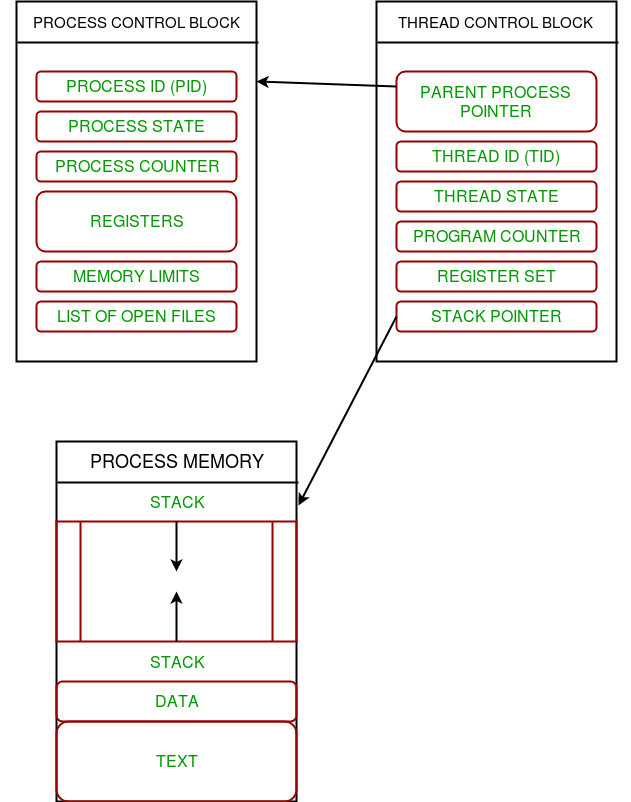Multiple threads can exist within one process where:

• Each thread contains its own register set and local variables (stored in stack).
• All thread of a process share global variables (stored in heap) and the program code.

Consider the diagram below to understand how multiple threads exist in memory: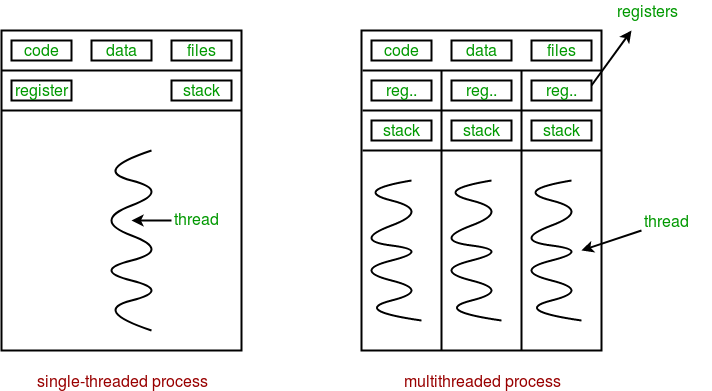Multithreading is defined as the ability of a processor to execute multiple threads concurrently.

In a simple, single-core CPU, it is achieved using frequent switching between threads. This is termed as context switching. In context switching, the state of a thread is saved and state of another thread is loaded whenever any interrupt (due to I/O or manually set) takes place. Context switching takes place so frequently that all the threads appear to be running parallely (this is termed as multitasking).

Consider the diagram below in which a process contains two active threads: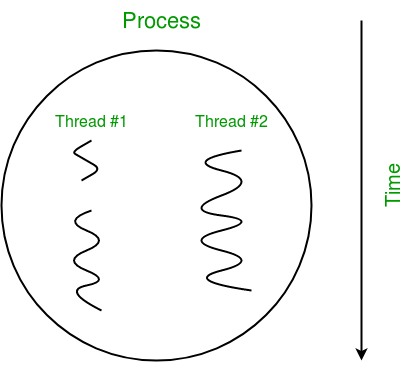In Python, the threading module provides a very simple and intuitive API for spawning multiple threads in a program.

Let us consider a simple example using threading module:

 `# Python program to illustrate the concept` `# of threading` `# importing the threading module` `import` `threading` ` ` `def` `print_cube(num):` `    ``"""` `    ``function to print cube of given num` `    ``"""` `    ``print``(``"Cube: {}"``.``format``(num ``*` `num ``*` `num))` ` ` `def` `print_square(num):` `    ``"""` `    ``function to print square of given num` `    ``"""` `    ``print``(``"Square: {}"``.``format``(num ``*` `num))` ` ` `if` `__name__ ``=``=` `"__main__"``:` `    ``# creating thread` `    ``t1 ``=` `threading.Thread(target``=``print_square, args``=``(``10``,))` `    ``t2 ``=` `threading.Thread(target``=``print_cube, args``=``(``10``,))` ` ` `    ``# starting thread 1` `    ``t1.start()` `    ``# starting thread 2` `    ``t2.start()` ` ` `    ``# wait until thread 1 is completely executed` `    ``t1.join()` `    ``# wait until thread 2 is completely executed` `    ``t2.join()` ` ` `    ``# both threads completely executed` `    ``print``(``"Done!"``)`
```Square: 100
Cube: 1000
Done!
```

Let us try to understand the above code:

• To import the threading module, we do:
```import threading
```
• To create a new thread, we create an object of Thread class. It takes following arguments:
• target: the function to be executed by thread
• args: the arguments to be passed to the target function

In above example, we created 2 threads with different target functions:

```t1 = threading.Thread(target=print_square, args=(10,))
```
• To start a thread, we use start method of Thread class.
```t1.start()
t2.start()
```
• Once the threads start, the current program (you can think of it like a main thread) also keeps on executing. In order to stop execution of current program until a thread is complete, we use join method.
```t1.join()
t2.join()
```

As a result, the current program will first wait for the completion of t1 and then t2. Once, they are finished, the remaining statements of current program are executed.

Consider the diagram below for a better understanding of how above program works: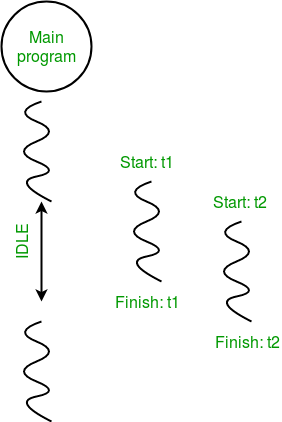Consider the python program given below in which we print thread name and corresponding process for each task:

 `# Python program to illustrate the concept` `# of threading` `import` `threading` `import` `os` ` ` `def` `task1():` `    ``print``(``"Task 1 assigned to thread: {}"``.``format``(threading.current_thread().name))` `    ``print``(``"ID of process running task 1: {}"``.``format``(os.getpid()))` ` ` `def` `task2():` `    ``print``(``"Task 2 assigned to thread: {}"``.``format``(threading.current_thread().name))` `    ``print``(``"ID of process running task 2: {}"``.``format``(os.getpid()))` ` ` `if` `__name__ ``=``=` `"__main__"``:` ` ` `    ``# print ID of current process` `    ``print``(``"ID of process running main program: {}"``.``format``(os.getpid()))` ` ` `    ``# print name of main thread` `    ``print``(``"Main thread name: {}"``.``format``(threading.current_thread().name))` ` ` `    ``# creating threads` `    ``t1 ``=` `threading.Thread(target``=``task1, name``=``'t1'``)` `    ``t2 ``=` `threading.Thread(target``=``task2, name``=``'t2'``)  ` ` ` `    ``# starting threads` `    ``t1.start()` `    ``t2.start()` ` ` `    ``# wait until all threads finish` `    ``t1.join()` `    ``t2.join()`
```ID of process running main program: 11758
ID of process running task 1: 11758
ID of process running task 2: 11758
```

Let us try to understand the above code:

• We use os.getpid() function to get ID of current process.
```print("ID of process running main program: {}".format(os.getpid()))
```

As it is clear from the output, the process ID remains same for all threads.

• We use threading.main_thread() function to get the main thread object. In normal conditions, the main thread is the thread from which the Python interpreter was started. name attribute of thread object is used to get the name of thread.
```print("Main thread name: {}".format(threading.main_thread().name))
```
```print("Task 1 assigned to thread: {}".format(threading.current_thread().name))
```

The diagram given below clears the above concept: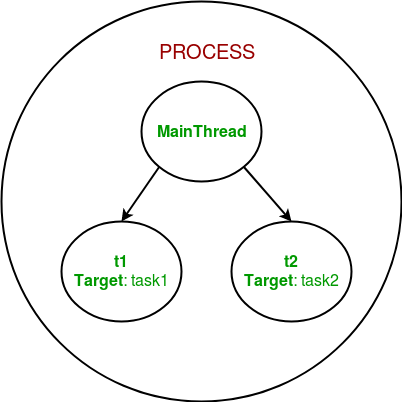So, this was a brief introduction to multithreading in Python. The next article in this series covers synchronization between multiple threads.

Now, let’s discusses the concept of thread synchronization in case of multithreading in Python programming language.

Thread synchronization is defined as a mechanism which ensures that two or more concurrent threads do not simultaneously execute some particular program segment known as critical section.

Critical section refers to the parts of the program where the shared resource is accessed.

For example, in the diagram below, 3 threads try to access shared resource or critical section at the same time.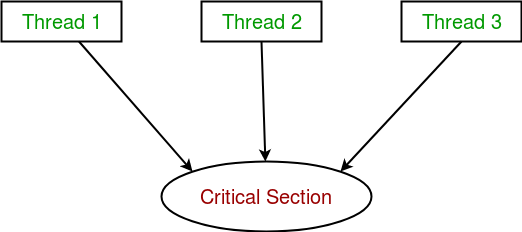Concurrent accesses to shared resource can lead to race condition.

A race condition occurs when two or more threads can access shared data and they try to change it at the same time. As a result, the values of variables may be unpredictable and vary depending on the timings of context switches of the processes.

Consider the program below to understand the concept of race condition:

 `import` `threading` ` ` `# global variable x` `x ``=` `0` ` ` `def` `increment():` `    ``"""` `    ``function to increment global variable x` `    ``"""` `    ``global` `x` `    ``x ``+``=` `1` ` ` `def` `thread_task():` `    ``"""` `    ``task for thread` `    ``calls increment function 100000 times.` `    ``"""` `    ``for` `_ ``in` `range``(``100000``):` `        ``increment()` ` ` `def` `main_task():` `    ``global` `x` `    ``# setting global variable x as 0` `    ``x ``=` `0` ` ` `    ``# creating threads` `    ``t1 ``=` `threading.Thread(target``=``thread_task)` `    ``t2 ``=` `threading.Thread(target``=``thread_task)` ` ` `    ``# start threads` `    ``t1.start()` `    ``t2.start()` ` ` `    ``# wait until threads finish their job` `    ``t1.join()` `    ``t2.join()` ` ` `if` `__name__ ``=``=` `"__main__"``:` `    ``for` `i ``in` `range``(``10``):` `        ``main_task()` `        ``print``(``"Iteration {0}: x = {1}"``.``format``(i,x))`

Output:

```Iteration 0: x = 175005
Iteration 1: x = 200000
Iteration 2: x = 200000
Iteration 3: x = 169432
Iteration 4: x = 153316
Iteration 5: x = 200000
Iteration 6: x = 167322
Iteration 7: x = 200000
Iteration 8: x = 169917
Iteration 9: x = 153589```

In above program:

• Two threads t1 and t2 are created in main_task function and global variable x is set to 0.
• increment function will increment the global variable x by 1 in each call.

The expected final value of x is 200000 but what we get in 10 iterations of main_task function is some different values.

This happens due to concurrent access of threads to the shared variable x. This unpredictability in value of x is nothing but race condition.

Given below is a diagram which shows how can race condition occur in above program: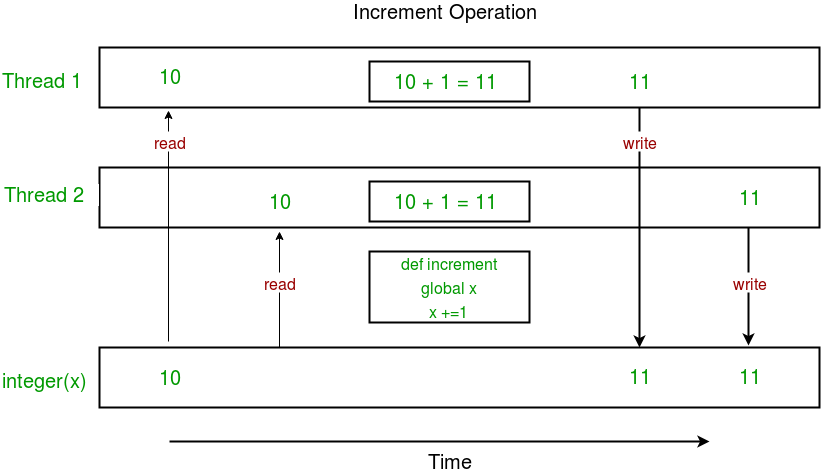Notice that expected value of x in above diagram is 12 but due to race condition, it turns out to be 11!

Hence, we need a tool for proper synchronization between multiple threads.

Using Locks

threading module provides a Lock class to deal with the race conditions. Lock is implemented using a Semaphore object provided by the Operating System.

A semaphore is a synchronization object that controls access by multiple processes/threads to a common resource in a parallel programming environment. It is simply a value in a designated place in operating system (or kernel) storage that each process/thread can check and then change. Depending on the value that is found, the process/thread can use the resource or will find that it is already in use and must wait for some period before trying again. Semaphores can be binary (0 or 1) or can have additional values. Typically, a process/thread using semaphores checks the value and then, if it using the resource, changes the value to reflect this so that subsequent semaphore users will know to wait.

Lock class provides following methods:

• acquire([blocking]) : To acquire a lock. A lock can be blocking or non-blocking.
• When invoked with the blocking argument set to True (the default), thread execution is blocked until the lock is unlocked, then lock is set to locked and return True.
• When invoked with the blocking argument set to False, thread execution is not blocked. If lock is unlocked, then set it to locked and return True else return False immediately.
• release() : To release a lock.
• When the lock is locked, reset it to unlocked, and return. If any other threads are blocked waiting for the lock to become unlocked, allow exactly one of them to proceed.

Consider the example given below:

 `import` `threading` ` ` `# global variable x` `x ``=` `0` ` ` `def` `increment():` `    ``"""` `    ``function to increment global variable x` `    ``"""` `    ``global` `x` `    ``x ``+``=` `1` ` ` `def` `thread_task(lock):` `    ``"""` `    ``task for thread` `    ``calls increment function 100000 times.` `    ``"""` `    ``for` `_ ``in` `range``(``100000``):` `        ``lock.acquire()` `        ``increment()` `        ``lock.release()` ` ` `def` `main_task():` `    ``global` `x` `    ``# setting global variable x as 0` `    ``x ``=` `0` ` ` `    ``# creating a lock` `    ``lock ``=` `threading.Lock()` ` ` `    ``# creating threads` `    ``t1 ``=` `threading.Thread(target``=``thread_task, args``=``(lock,))` `    ``t2 ``=` `threading.Thread(target``=``thread_task, args``=``(lock,))` ` ` `    ``# start threads` `    ``t1.start()` `    ``t2.start()` ` ` `    ``# wait until threads finish their job` `    ``t1.join()` `    ``t2.join()` ` ` `if` `__name__ ``=``=` `"__main__"``:` `    ``for` `i ``in` `range``(``10``):` `        ``main_task()` `        ``print``(``"Iteration {0}: x = {1}"``.``format``(i,x))`

Output:

```Iteration 0: x = 200000
Iteration 1: x = 200000
Iteration 2: x = 200000
Iteration 3: x = 200000
Iteration 4: x = 200000
Iteration 5: x = 200000
Iteration 6: x = 200000
Iteration 7: x = 200000
Iteration 8: x = 200000
Iteration 9: x = 200000
```

Let us try to understand the above code step by step:

• Firstly, a Lock object is created using:
```  lock = threading.Lock()
```
• Then, lock is passed as target function argument:
```  t1 = threading.Thread(target=thread_task, args=(lock,))
```
• In the critical section of target function, we apply lock using lock.acquire() method. As soon as a lock is acquired, no other thread can access the critical section (here, increment function) until the lock is released using lock.release() method.
```  lock.acquire()
increment()
lock.release()
```

As you can see in the results, the final value of x comes out to be 200000 every time (which is the expected final result).

Here is a diagram given below which depicts the implementation of locks in above program: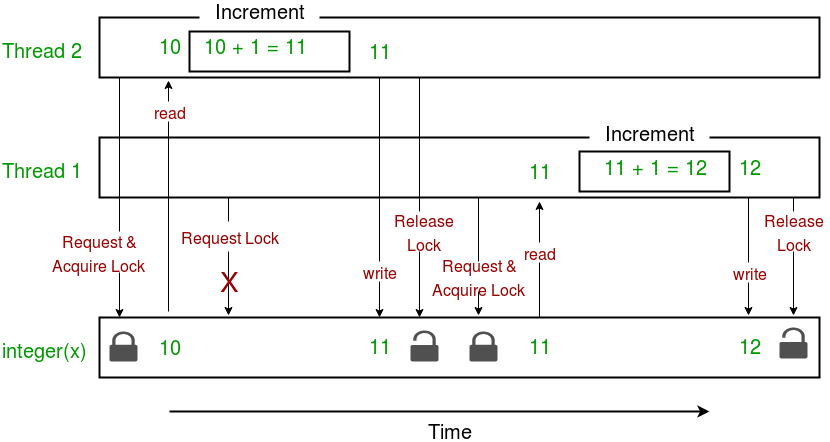This brings us to the end of this tutorial series on Multithreading in Python.

• It doesn’t block the user. This is because threads are independent of each other.
• Better use of system resources is possible since threads execute tasks parallely.
• Enhanced performance on multi-processor machines.

• As number of threads increase, complexity increases.
• Synchronization of shared resources (objects, data) is necessary.
• It is difficult to debug, result is sometimes unpredictable.
• Constructing and synchronizing threads is CPU/memory intensive.

This article is contributed by Nikhil Kumar. If you like GeeksforGeeks and would like to contribute, you can also write an article using contribute.geeksforgeeks.org or mail your article to contribute@geeksforgeeks.org. See your article appearing on the GeeksforGeeks main page and help other Geeks.

Last Updated on March 1, 2022 by admin

## Python PolymorphismPython Polymorphism

Polymorphism in Python What is Polymorphism: The word polymorphism means having many forms. In programming, polymorphism

## Iterating over rows & columns in Pandas DataFrameIterating over rows & columns in Pandas DataFrame

Iteration is a general term for taking each item of something, one after another. Pandas

## Python – Move or Copy Files and DirectoriesPython – Move or Copy Files and Directories

Python | Move or Copy Files and Directories Let’s say we want to copy or

## Python – os.path.basename() methodPython – os.path.basename() method

Python | os.path.basename() method OS module in Python provides functions for interacting with the operating system.

Python | Read csv using pandas.read_csv() Python is a great language for doing data analysis,

## SQL using PythonSQL using Python

SQL using Python In this article, integrating SQLite3 with Python is discussed. Here we will

## Turtle Programming in PythonTurtle Programming in Python

Turtle Programming in Python “Turtle” is a Python feature like a drawing board, which lets

## 3D Scatter Plotting in Python using Matplotlib3D Scatter Plotting in Python using Matplotlib

3D Scatter Plotting in Python using Matplotlib A 3D Scatter Plot is a mathematical diagram, the most